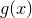## 10.6.1 The replot command revisited

In multiplot mode, the replot command can be used to modify the last plot added to the page. For example, the following would change the title of the latest plot to ‘foo’, and add a plot of the functionto it:

```set title 'foo'
replot cos(x)
```

Additionally, it is possible to modify any plot on the page by adding an item modifier to the replot statement to specify which plot should be replotted. The following example would produce two plots, and then add an additional function to the first plot:

```set multiplot
plot f(x)
set origin 10,0
plot g(x)
replot item 1 h(x)
```

If no item number is specified, then the replot command acts by default upon the most recent plot to have been added to the multiplot canvas.